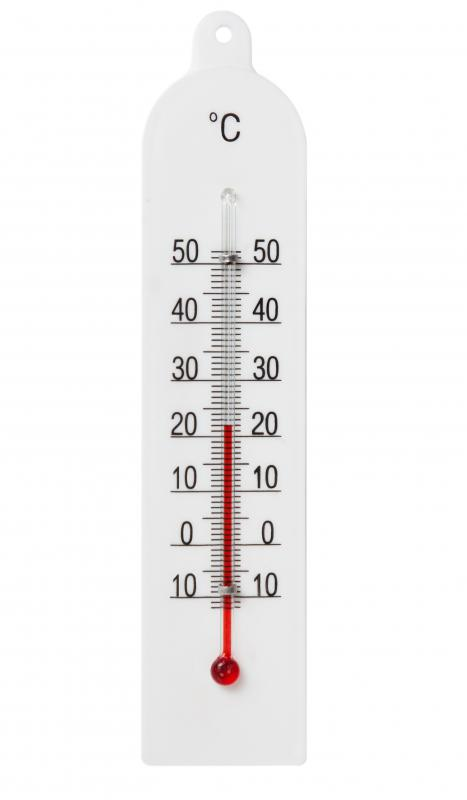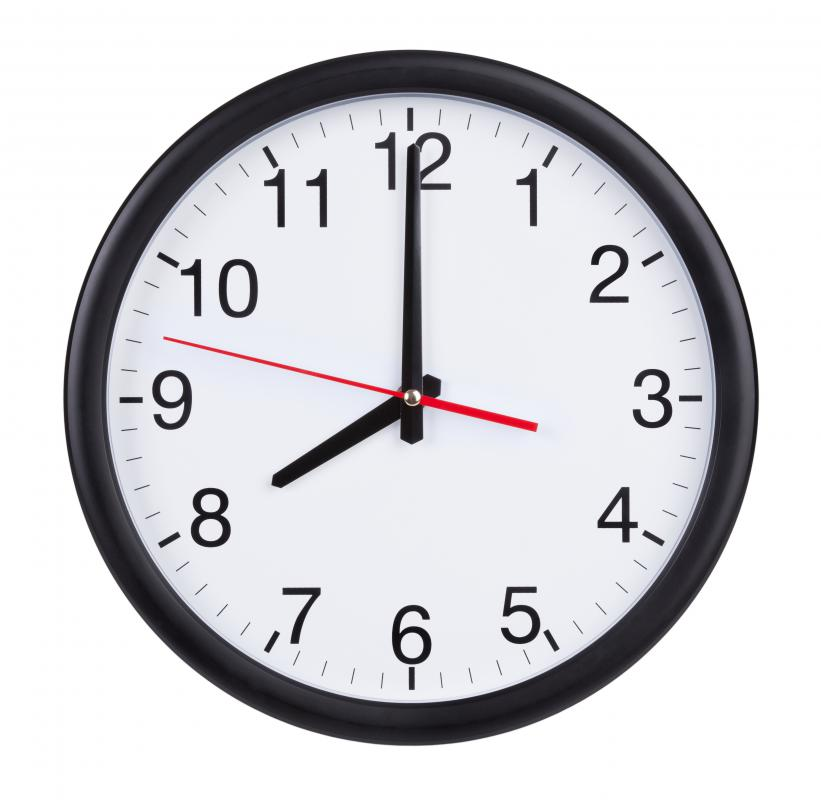# What are Continuous Variables? (with pictures)

Victoria Blackburn
Victoria BlackburnTemperature can have an infinite number of values between two points.

Variable is a term used to describe something that can be measured and can also vary. The opposite of a variable is a constant. A constant is a quantity that doesn’t change within a specific context. In scientific experiments, variables are used as a way to group the data together. Variables can be grouped as either discrete or continuous variables.The seconds hand on a clock is in continuous motion.

Generally, variables are characteristics of a group of objects or events that can be measured over a number of different numerical values. Discrete variables can have only a certain number of different values between two given points. For example, in a family, there can be one, two, or three children, but there cannot be a continuous scale of 1.1, 1.5, or 1.75 children.

Continuous variables can have an infinite number of different values between two given points. As shown above, there cannot be a continuous scale of children within a family. If height were being measured though, the variables would be continuous as there are an unlimited number of possibilities even if only looking at between 1 and 1.1 meters.

It is important to remember that both kinds of variables are so grouped based on the scale used to measure them and what is being measured. In most scientific experiments, a discrete scale is used to measure both kinds of variables. Because there are an infinite amount of possibilities, this means the measurements of continuous variables are often rounded off to make the data easier to work with.

Both discrete and continuous variables can take on one of two roles in a scientific experiment. During an experiment, the scientist often wants to observe the results of changing one variable. Only one variable is often changed, as it would be difficult to determine what had caused the relevant response if multiple variables were influenced.

The variable that is manipulated by the scientist is the independent variable, while the dependent variable is the one that responds to the change. In other words, the response of one variable is dependent on the changes to the other variable. If there were no change initially to any of the variables, then there wouldn’t be a response by the dependent variable.

For example, during an experiment, the amount of light shining on a plant is changed. The amount of light would be the independent variable. To make measurements that can be repeated, the independent variable is likely to be a discrete variable, such as one hour, two hours, or three hours of light. The response of the plant, how much it grows or the direction it grows, will be the dependent variable. As the amount the plant grows can be an infinite number of results, it is a dependent continuous variable.

## You might also Like## Discussion Comments

anon161471

this article gave me lots of understanding on the subject matter! Thanks to you.

anon154233

quite good advice although i didn't understand some of the terms used, but thanks.

anon142999

literally the clearest explanation I've found. many thanks.

anon116549

This article helped me a lot. Thanks

Forgot password?
###### Register:
•Temperature can have an infinite number of values between two points.
•The seconds hand on a clock is in continuous motion.